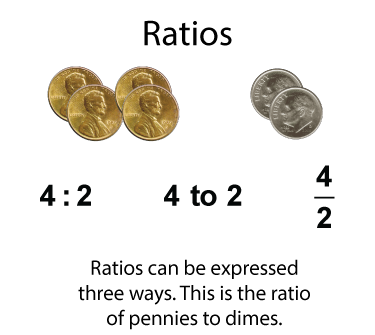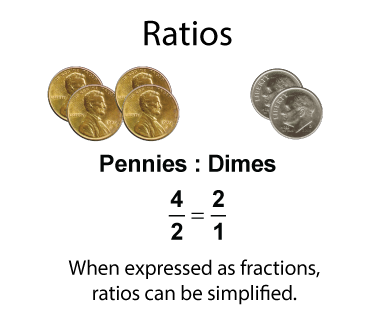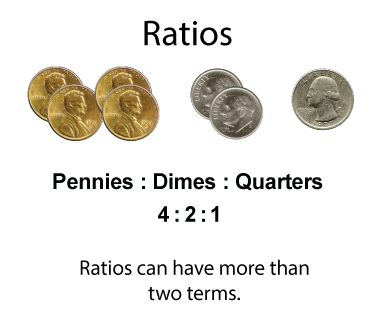# Video Transcript: Ratios: What Are Ratios?

## What Are Ratios?

A ratio is the relationship between two or more quantities among a group of items. Let's look at an example.Here is a collection of coins. The ratio of pennies to dimes can be expressed as a ratio, and a ratio can be written in three different, but equivalent ways.

When expressed as fractions, ratios can then be rewritten as fractions in simplest form.For some groupings, you can write a ratio that includes three terms.Ratios usually include similar types of objects. Here is a collection of different types of balls.Rates are a special type of ratio. Rates involve different types of quantities. Here is an example of a rate involving cost and weight.This is the transcript that goes with the video segment entitled Video Tutorial: Ratios: What Are They?